Activate JavaScript to enable computations!

# Pulsed Power Toolbox

This is the start of a collection of Pulsed Power related formulas and calculators that I found usefull. However, whenever I could find resources on the internet already, I tried to refer to them rather than to re-invent the wheel. Consequently you might also find some of the links provided helpful.

 Formulas for the Calculation of Common Inductances*) (unless otherwise noted use SI-units in all presented formulas) *) Planar geometries which are not very common in pulsed power systems are not covered in this table. Readers are refered to references 1, 6 and especially 8. More information on rectangular loops can be found here:http://www.qsl.net/in3otd/rlsim.html Of course no guarantee is given for the accuracy of the listed formulaes or the calculators although I tried my best to select the formulas carefully from the literature. Please let me know when you find any mistakes.

low frequency
inductance

formula

example

long, thin wire
(as segment of closed circuit)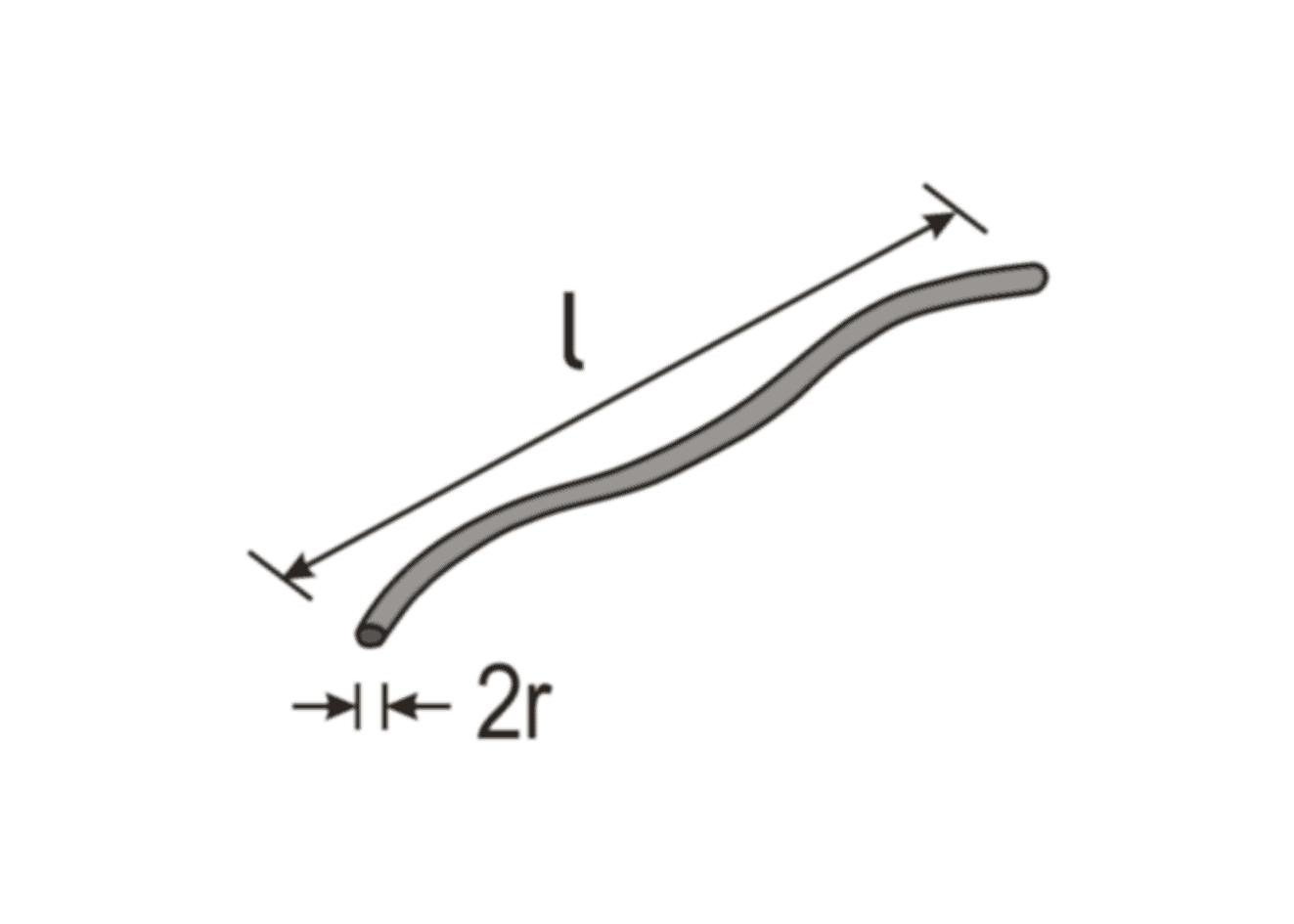(reference 1)

 r = mm l = cm nH

conductor from parallel wires
(currents in same direction)
r << d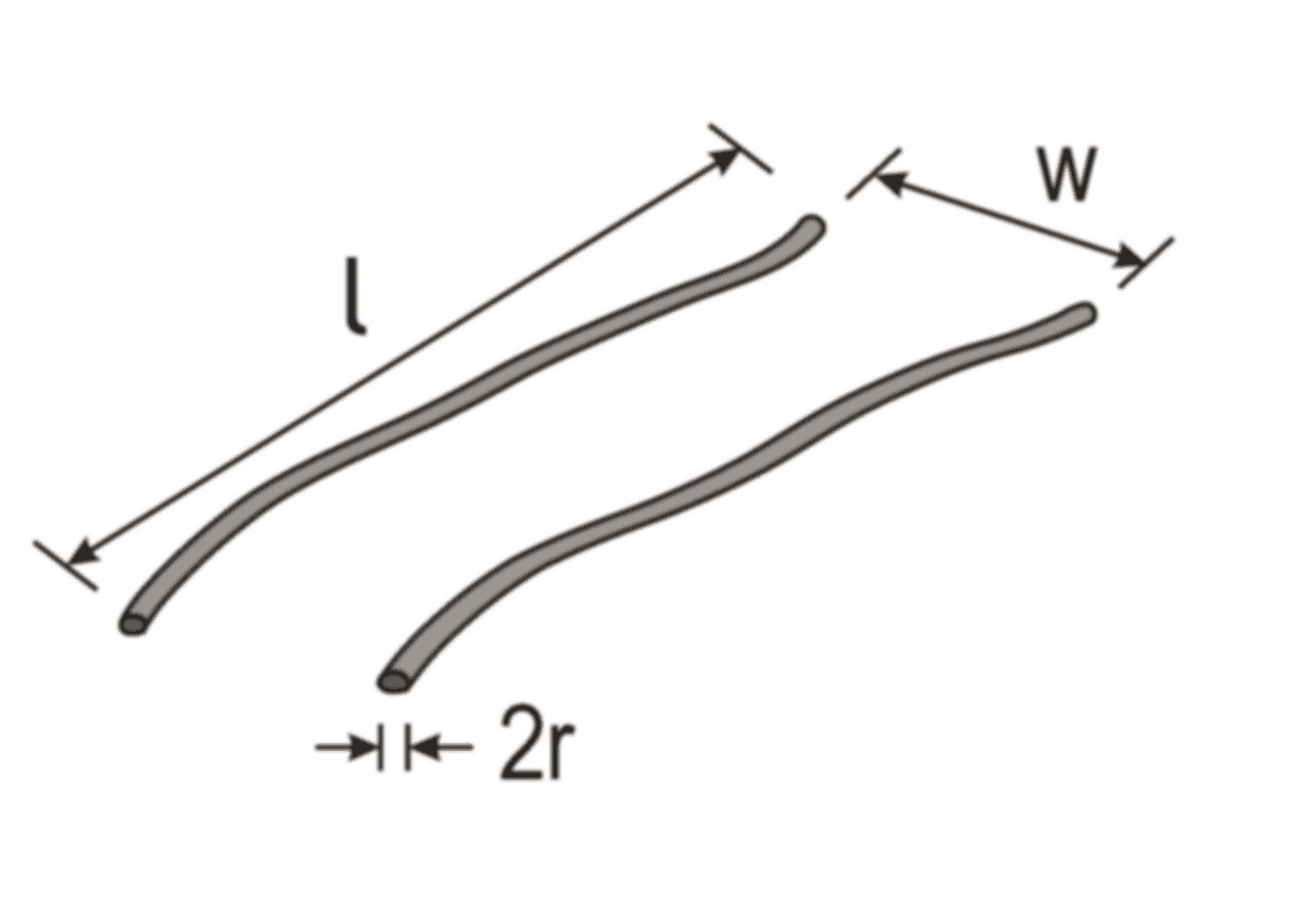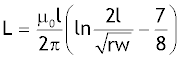(reference 8)

 l = cm w = mm r = mm nH

return circuit of parallel wires
(currents in opposite directions)
r << d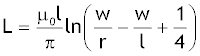(reference 1)
 l = 100 cm w = 2 cm r = 0.5 mm L = 1.478 μH

strip line
(as segment of closed circuit)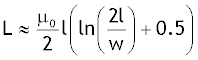(reference 1)
 l = 100 cm w = 50 cm L = 1.185 μH

return circuit of parallel plates
(current runs in opposite directions)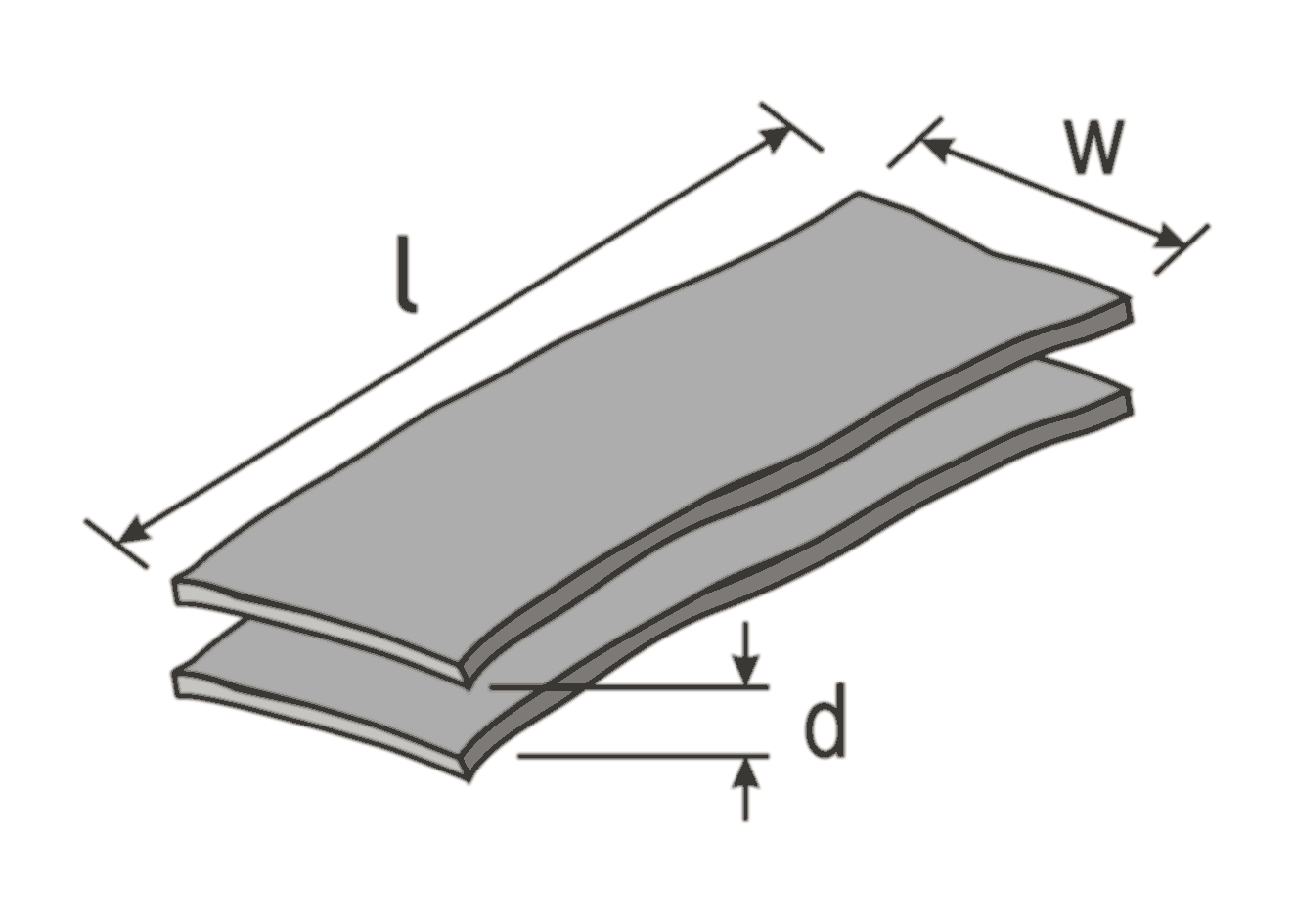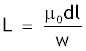(reference 2)

 l = cm w = mm d = mm nH

coaxial cable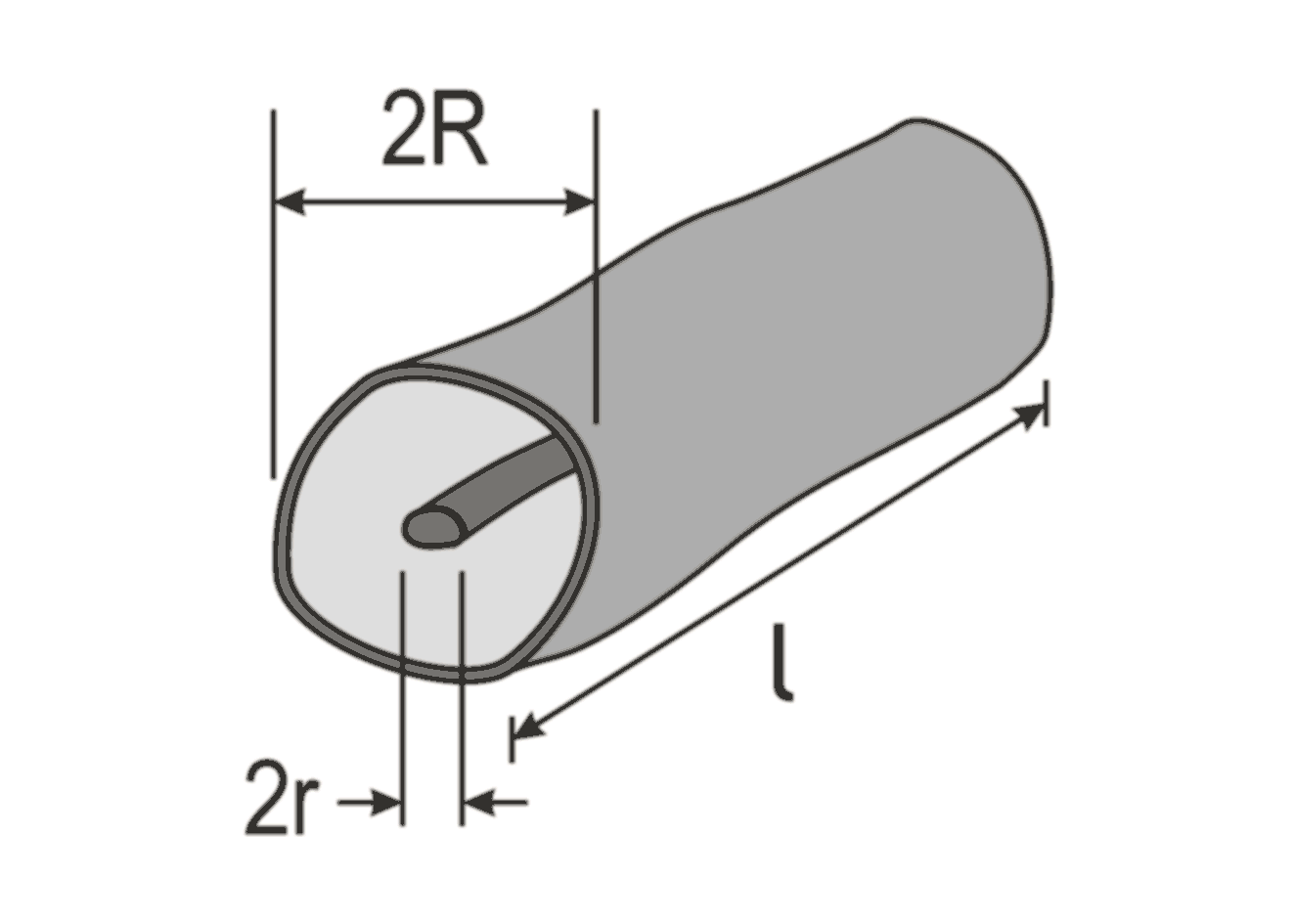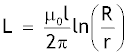(reference 2)

 l = cm R = mm r = mm nH

loop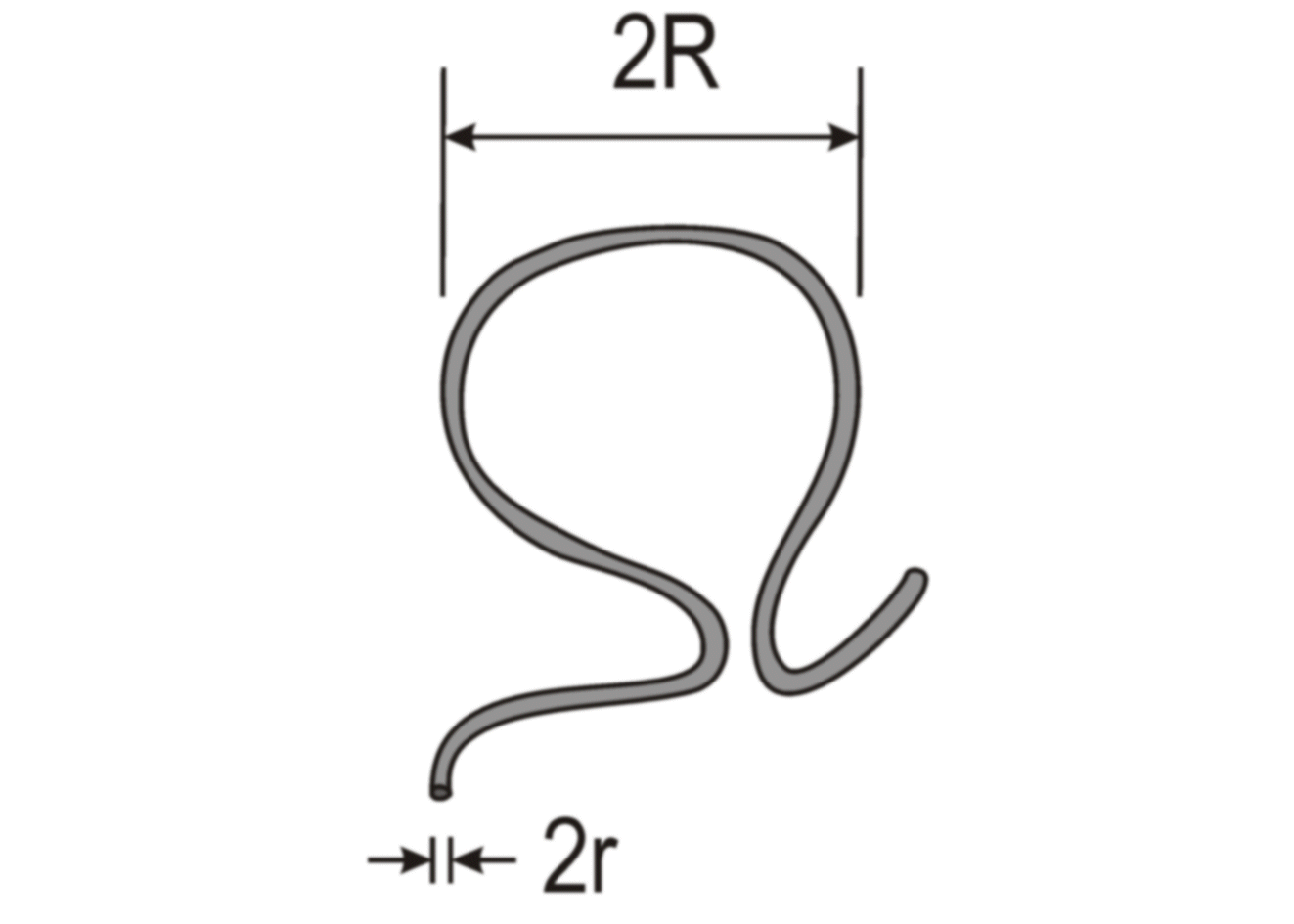(reference 1)
 R = mm r = mm nH

single layer solenoid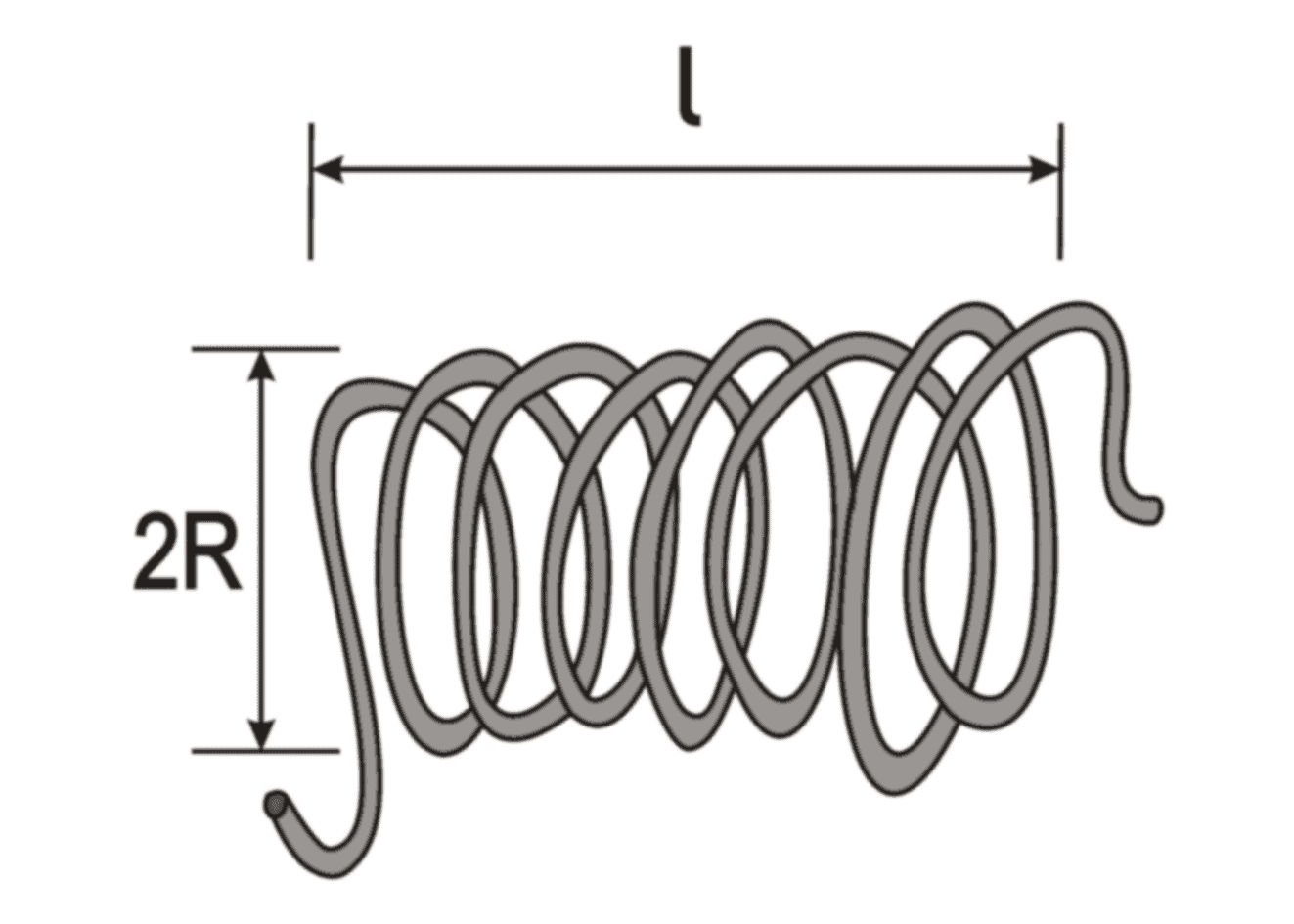N: number of turns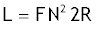"F-factor" is computed automatically

(reference 7)

The displayed equation is valid for dimensions that are entered in inches, which is taken into account in the provided calculation.
 R = mm l = cm N = μH

"Where great accuracy is required, a correction factor may be applied to the equation to take account of the fact that the coil is wound of spaced round wires rather than with a uniform current sheet. This correction rarely exceeds 0.5%, is greater for widely spaced turns, and increases with the number of turns." (see reference 7 for details)

single layer short solenoid
(l < 0.8 R)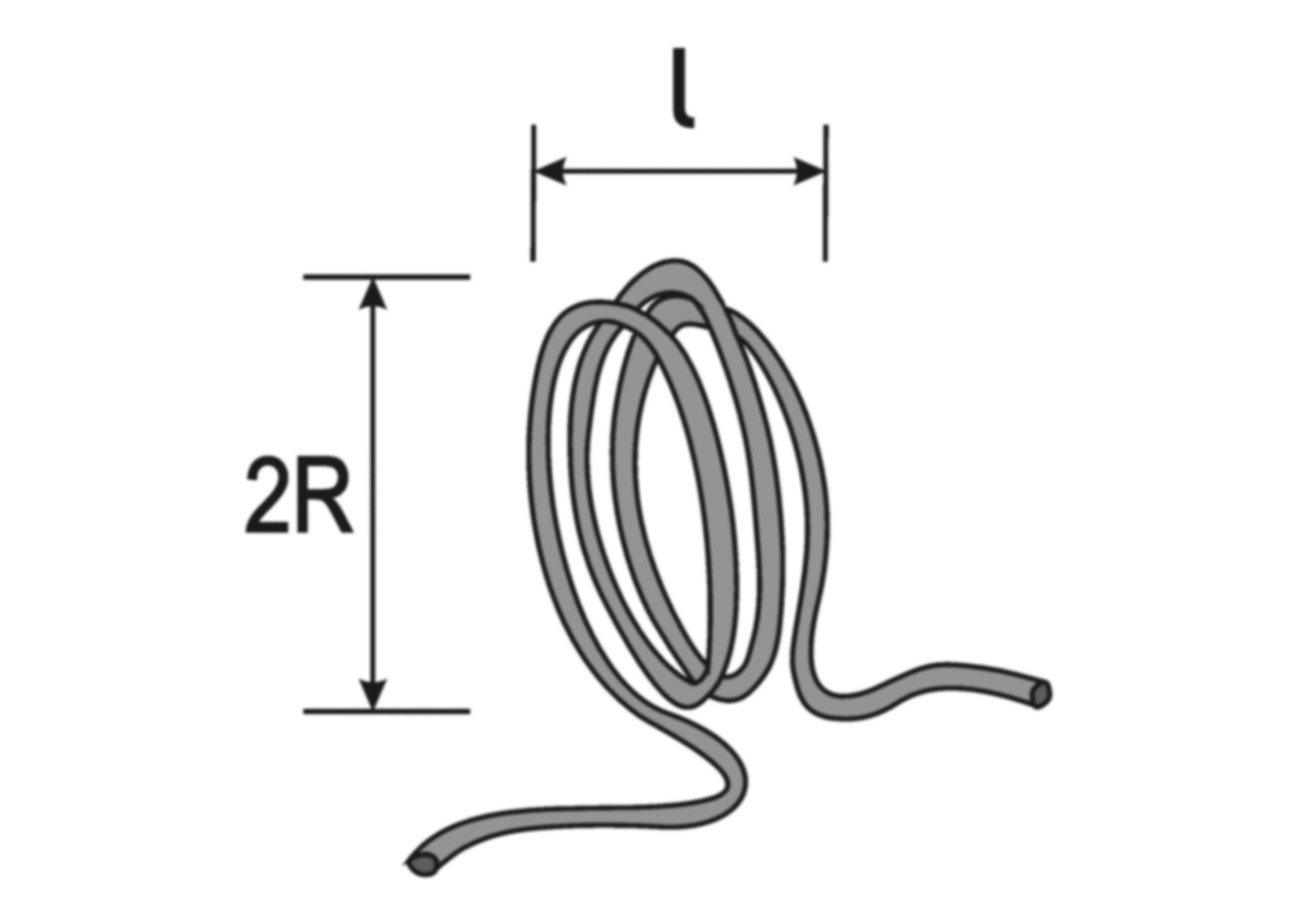N: number of turns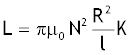Nagaoka-factor K is computed automatically

(reference 1)

 R = mm l = cm N = μH

single layer long solenoid
(l > 0.8 R)N: number of turns(reference 1)
 R = mm l = cm N = μH

single layer very long (ideal) solenoid
(l >> 2R
)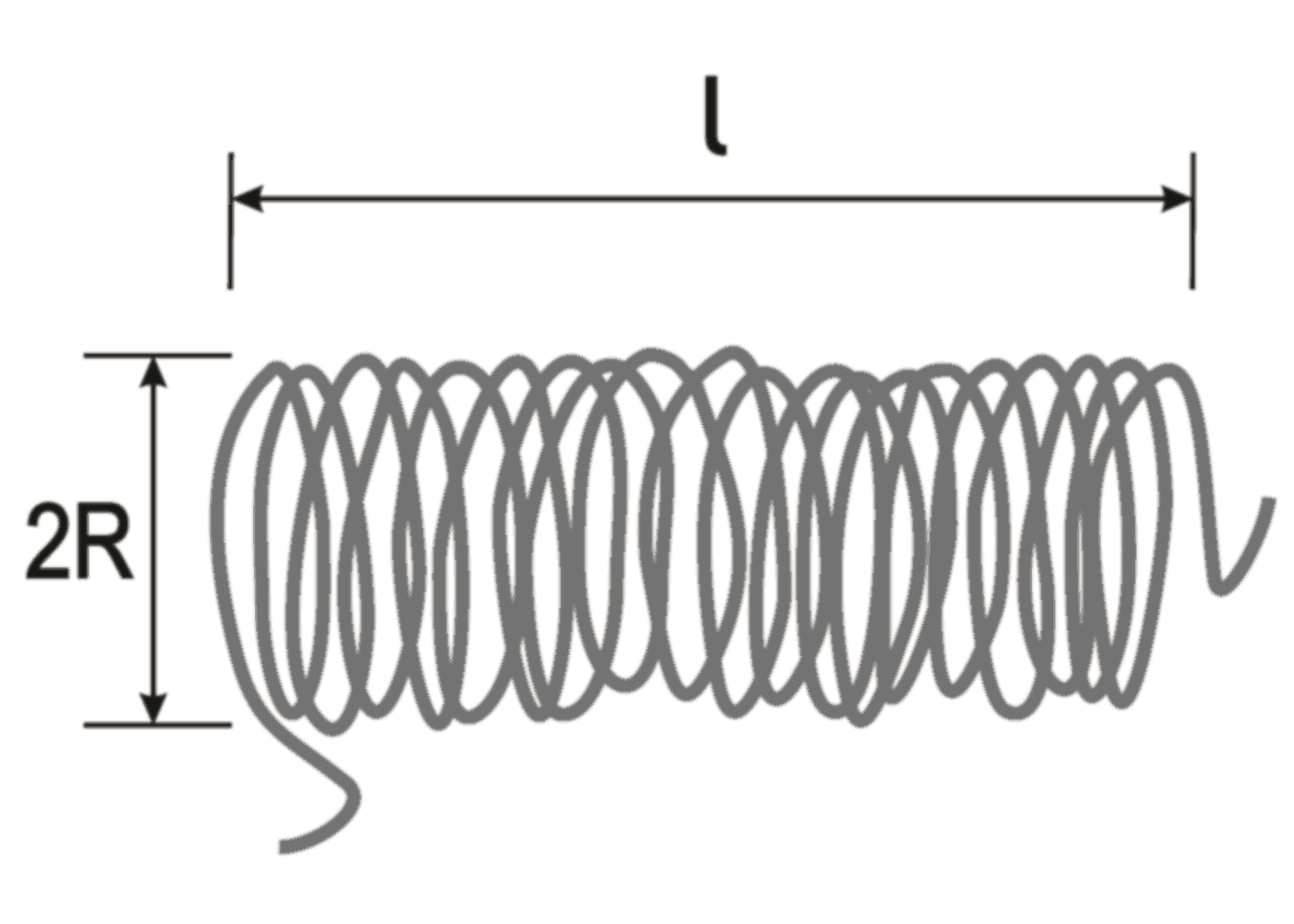N: number of turns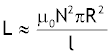(reference 3)

 R = mm l = cm N = μH

multi layer solenoid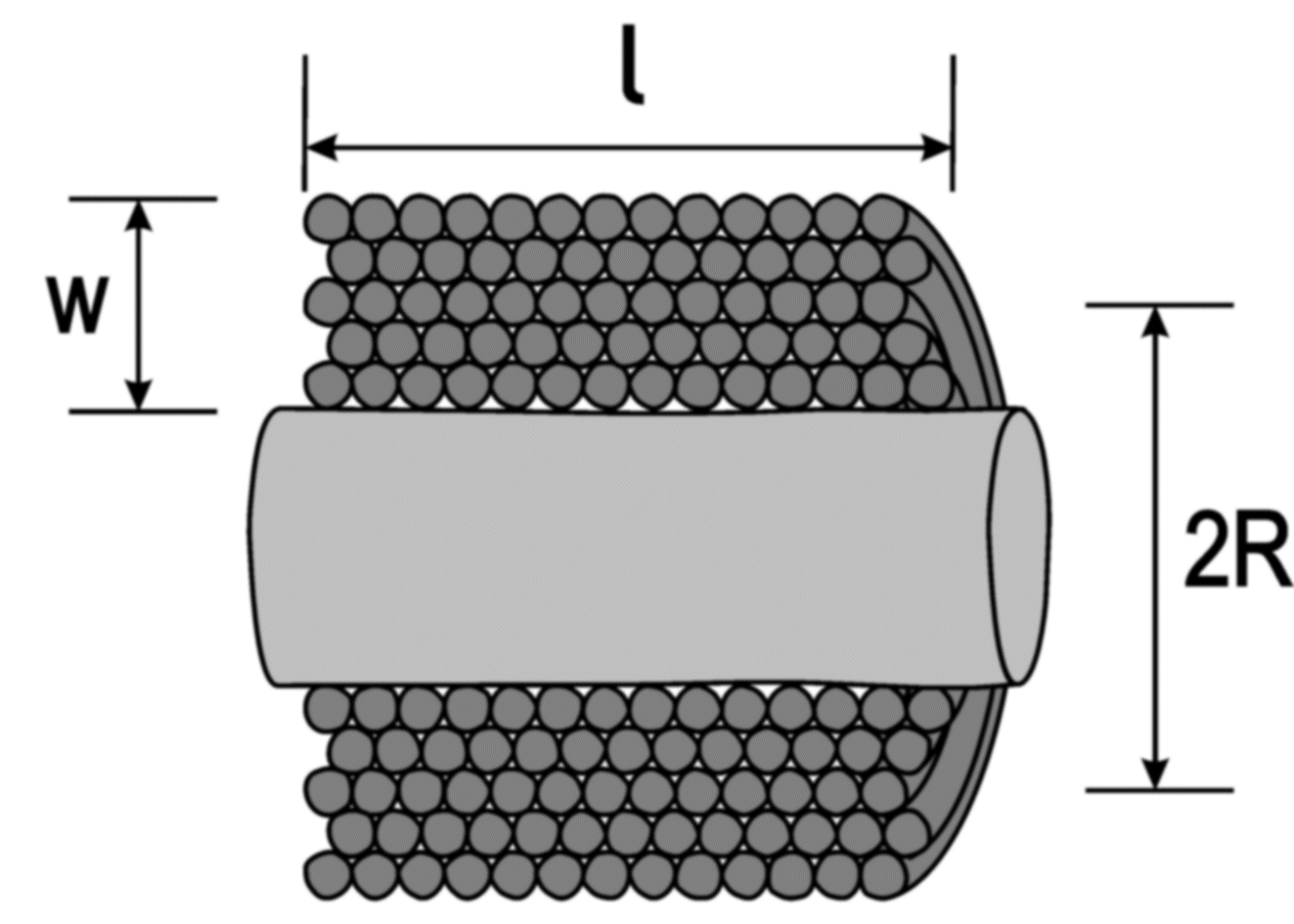N: number of turns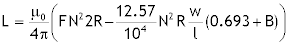"F-factor" and correction factor B are computed automatically

correction factor dL for insulation thickness: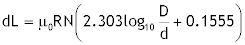D : distance between wire centers
d : diameter of bare wire

(reference 9)

 R = mm l = cm w = mm N = μH
 R = mm N = D = mm d = mm μH

multi layer thin wall solenoid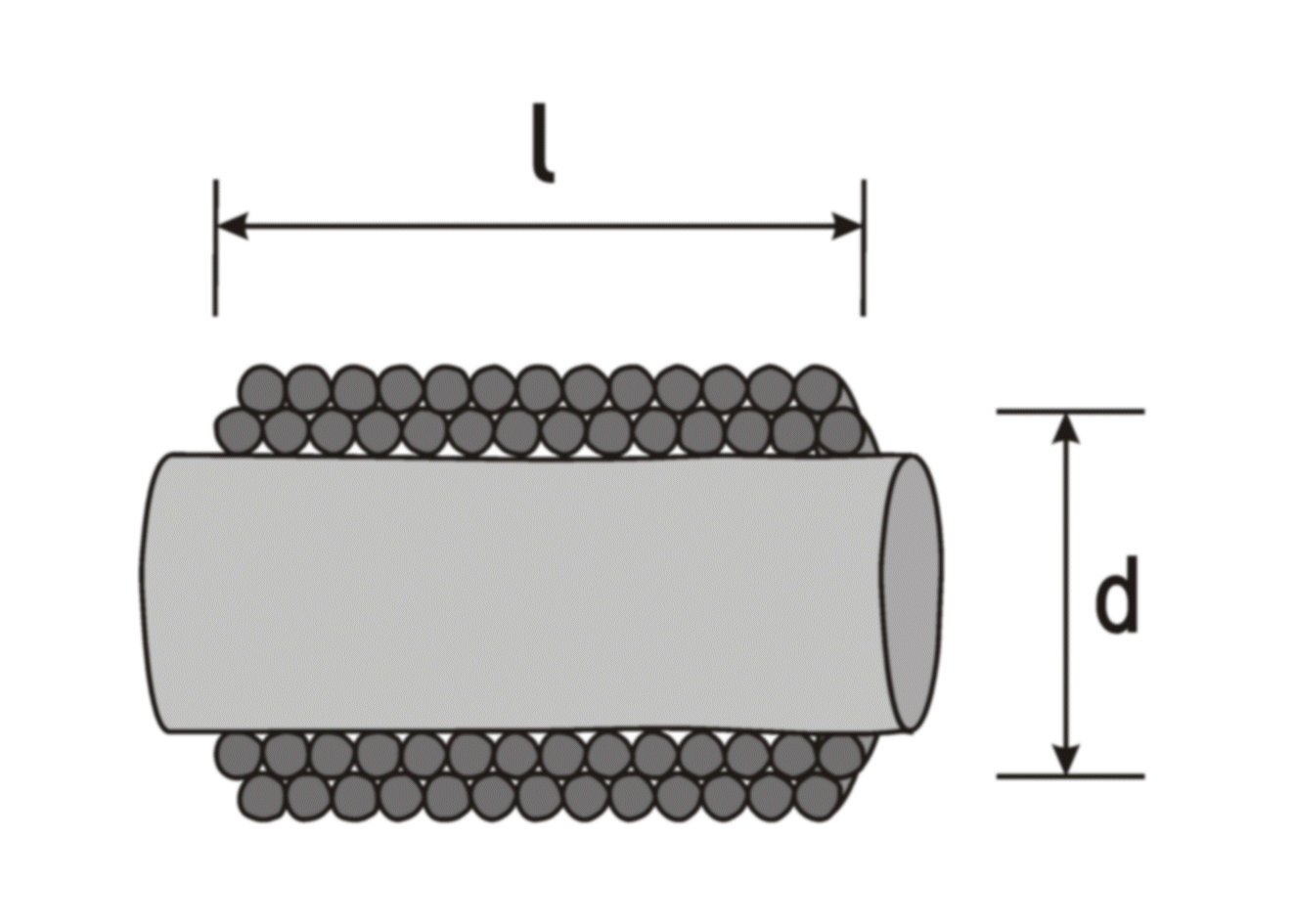N: number of turns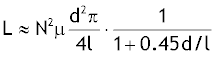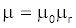(reference 4)
 N = 1000 d = 10 mm l = 5 cm mr = 1 L = 1.645 mH

toroid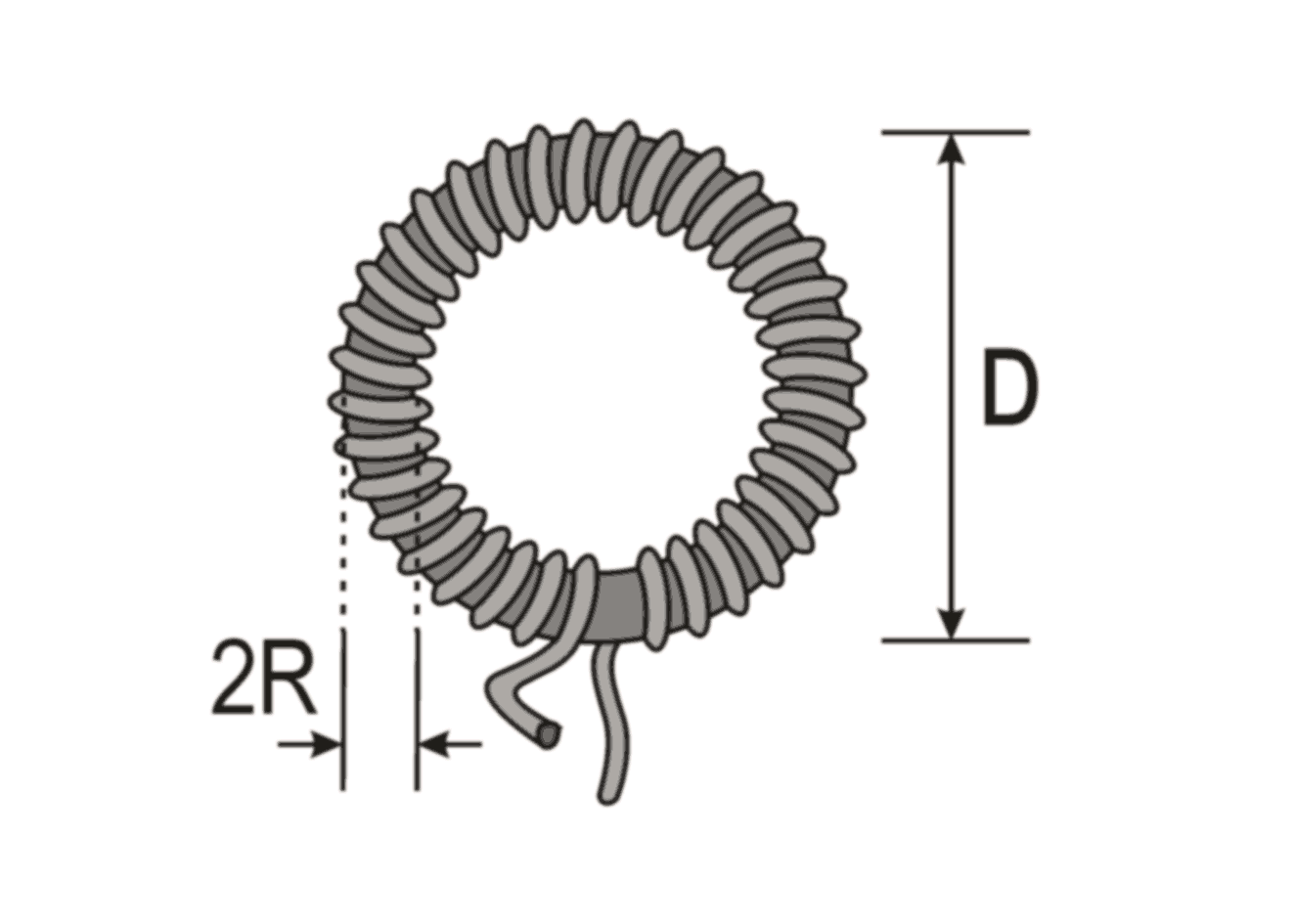N: number of turns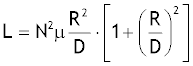(reference 4)

 N = R = mm D = cm mr = μH

spiral (flat coil)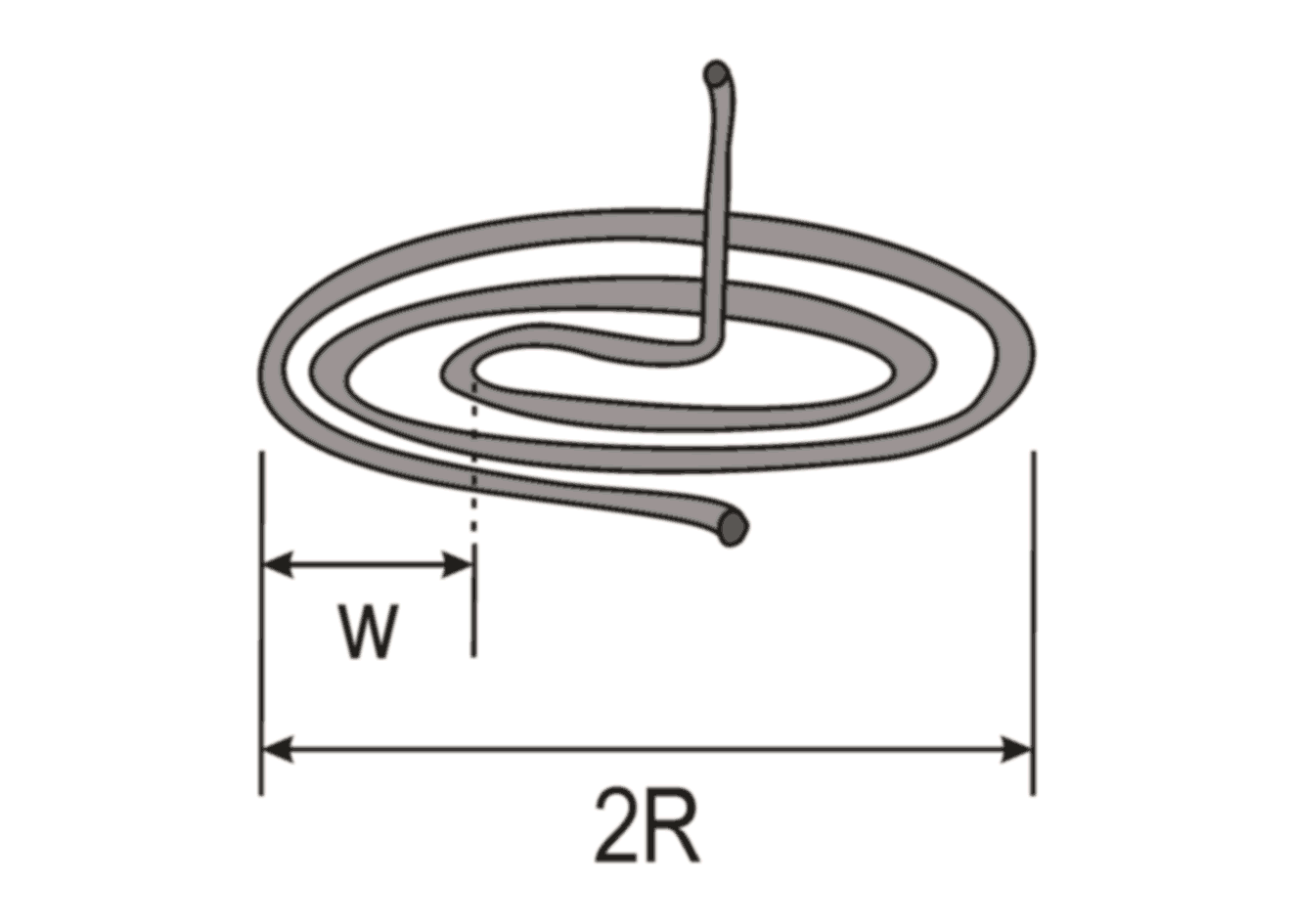N: number of turns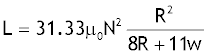(Wheeler formula)

(reference 1)
 N = 20 R = 5 cm w = 2 cm L = 63.501 μH
windings cover entire area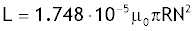(Schieber formula)

(reference 1)

 N = 20 R = 5 cm L = 1.38 nH

Both formulas can also be used for printed flat coils. For the Wheeler formulas errors up to 20% have been reported. A more accurate calculation takes the ratio between coil radius, R, and winding-thickness, w, into account (Grover method). See reference 1 for details.

conical coils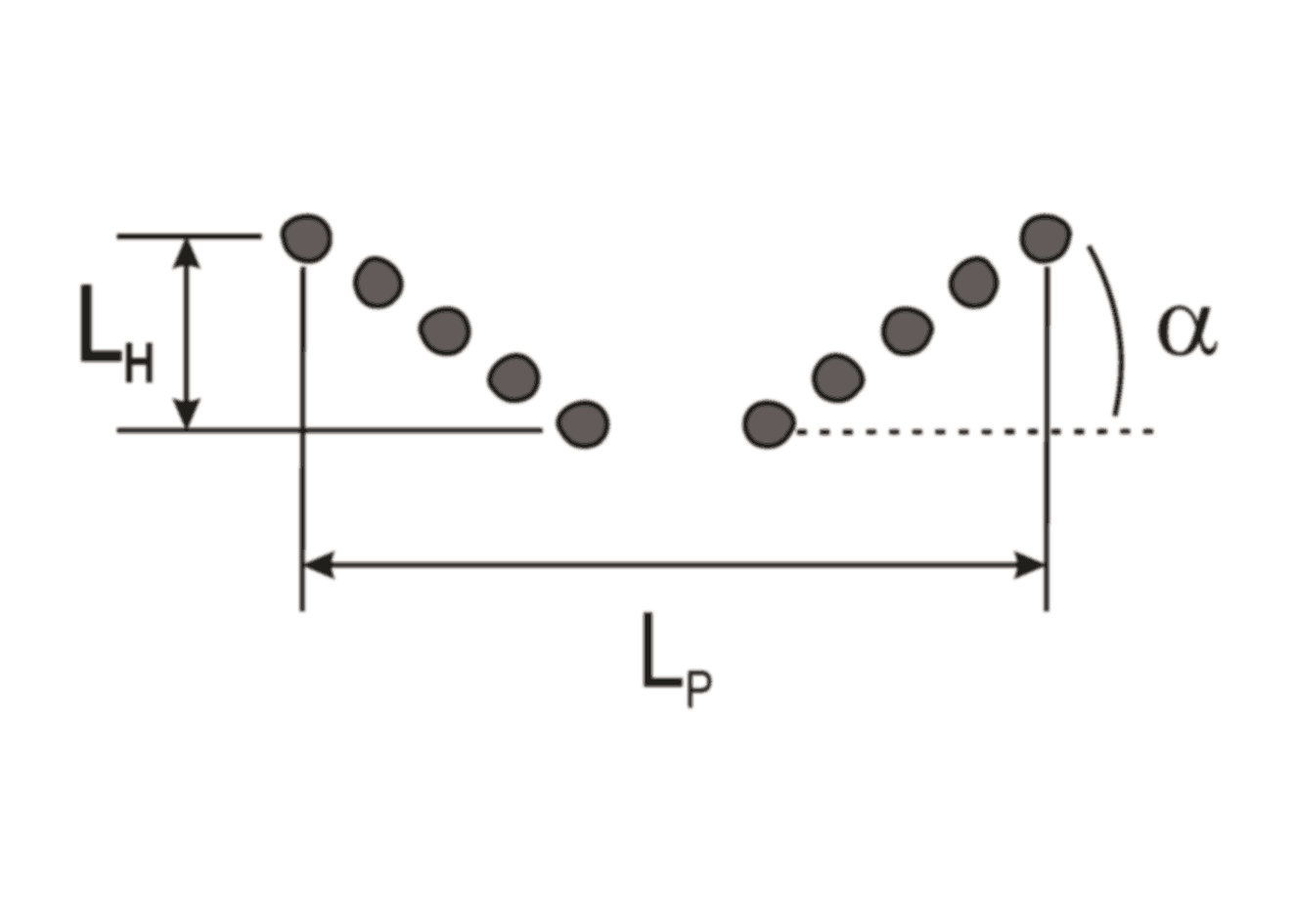The inductance of conical coils is calculated from the geometric sum of the helical and the planar contribution. See reference 5 for details.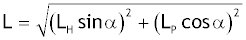LH : inductance of equivalent helical coil
LP : inductance of equivalent planar coil

(reference 5)

 References:     1) F.W. Grover, Inductance Calculations, Dover Publications, Mineola, 2004.     2) home.san.rr.com/nessengr/techdata/inductance/induc.html     3) H. Stöcker,Taschenbuch der Physik, Verlag Harri Deutsch, Frankfurt/Main, 1994, p. 334 (f = 1).     4) Formelsammlung Passive Bauelemente, www.iwe.uni-karlsruhe.de/download/WWW_PB_WET_FS.pdf     5) Herb's Tesla Page (Design Page), http://home.wtal.de/herbs_teslapage/design.html#des-2pri     6) University of Missouri-Rolla, Electromagnetic Compatibility Laboratory, www.emclab.umr.edu/new-induct, 2001.     7) F.E. Terman, Radio Engineers' Handbook, McGraw-Hill, New York, 1943, p.53.     8) F.W. Grover, Inductance Calculations, Dover Publications, Mineola, 2004, p.37.     9) F.E. Terman, Radio Engineers' Handbook, McGraw-Hill, New York, 1943, p.60.